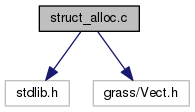GRASS Programmer's Manual  6.5.svn(2014)-r66266
struct_alloc.c File Reference
`#include <stdlib.h>`
`#include <grass/Vect.h>`
Include dependency graph for struct_alloc.c:Go to the source code of this file.

## Functions

P_NODE * dig_alloc_node ()

int dig_node_alloc_line (P_NODE *node, int add)

int dig_alloc_nodes (struct Plus_head *Plus, int add)

P_LINE * dig_alloc_line ()

int dig_alloc_lines (struct Plus_head *Plus, int add)

int dig_alloc_areas (struct Plus_head *Plus, int add)

int dig_alloc_isles (struct Plus_head *Plus, int add)

P_AREA * dig_alloc_area ()

P_ISLE * dig_alloc_isle ()

int dig_alloc_points (struct line_pnts *points, int num)

int dig_alloc_cats (struct line_cats *cats, int num)

int dig_area_alloc_line (P_AREA *area, int add)

int dig_area_alloc_isle (P_AREA *area, int add)

int dig_isle_alloc_line (P_ISLE *isle, int add)

int dig_out_of_memory ()

## Function Documentation

 P_AREA* dig_alloc_area ( )

Definition at line 189 of file struct_alloc.c.

References NULL.

Referenced by dig_add_area(), and dig_Rd_P_area().

 int dig_alloc_areas ( struct Plus_head * Plus, int add )

Definition at line 149 of file struct_alloc.c.

References NULL, and tools::size.

Referenced by dig_add_area(), and dig_load_plus().

 int dig_alloc_cats ( struct line_cats * cats, int num )

Definition at line 272 of file struct_alloc.c.

References dig__alloc_space(), and dig_out_of_memory().

Referenced by Vect_cat_set().

 P_ISLE* dig_alloc_isle ( )

Definition at line 211 of file struct_alloc.c.

References NULL.

Referenced by dig_add_isle(), and dig_Rd_P_isle().

 int dig_alloc_isles ( struct Plus_head * Plus, int add )

Definition at line 171 of file struct_alloc.c.

References G_debug(), NULL, and tools::size.

Referenced by dig_add_isle(), and dig_load_plus().

 P_LINE* dig_alloc_line ( )

Definition at line 110 of file struct_alloc.c.

References NULL.

Referenced by dig_Rd_P_line().

 int dig_alloc_lines ( struct Plus_head * Plus, int add )

Definition at line 127 of file struct_alloc.c.

References NULL, and tools::size.

Referenced by dig_add_line(), and dig_load_plus().

 P_NODE* dig_alloc_node ( )

Definition at line 40 of file struct_alloc.c.

References NULL.

Referenced by dig_add_node(), and dig_Rd_P_node().

 int dig_alloc_nodes ( struct Plus_head * Plus, int add )

Definition at line 93 of file struct_alloc.c.

References NULL, and tools::size.

Referenced by dig_add_node(), and dig_load_plus().

 int dig_alloc_points ( struct line_pnts * points, int num )

Definition at line 232 of file struct_alloc.c.

References dig__alloc_space(), and dig_out_of_memory().

 int dig_area_alloc_isle ( P_AREA * area, int add )

Definition at line 325 of file struct_alloc.c.

References G_debug(), NULL, and num.

Referenced by dig_area_add_isle(), and dig_Rd_P_area().

 int dig_area_alloc_line ( P_AREA * area, int add )

Definition at line 303 of file struct_alloc.c.

References NULL, and num.

Referenced by dig_add_area(), and dig_Rd_P_area().

 int dig_isle_alloc_line ( P_ISLE * isle, int add )

Definition at line 348 of file struct_alloc.c.

References G_debug(), NULL, and num.

Referenced by dig_add_isle(), and dig_Rd_P_isle().

 int dig_node_alloc_line ( P_NODE * node, int add )

Definition at line 63 of file struct_alloc.c.

References G_debug(), NULL, and num.

Referenced by dig_node_add_line(), and dig_Rd_P_node().

 int dig_out_of_memory ( )

Definition at line 369 of file struct_alloc.c.

Referenced by dig_alloc_cats(), dig_alloc_points(), and dig_build_area_with_line().